﻿ 膜系统下的一种多目标优化算法
«上一篇文章快速检索 高级检索

 智能系统学报2017, Vol. 12Issue (5): 678-683  DOI: 10.11992/tis.2017060130

### 引用本文TU Chuanyun, CHEN Taowei, YU Yimin, et al. Multi-objective optimization algorithm based on membrane system[J]. CAAI Transactions on Intelligent Systems, 2017, 12(5): 678-683. DOI: 10.11992/tis.201706013.### 文章历史

Multi-objective optimization algorithm based on membrane system
TU Chuanyun, CHEN Taowei, YU Yimin, ZHAO Kun
College of Information, Yunnan University of Finance and Economics, Kunming 650221, China
Abstract: In this paper, we propose a multi-objective optimization algorithm based on the theory of membrane optimization. Inspired by membrane computing, this algorithm combines membrane structure, multiple sets, and reaction rules to solve multi-objective optimization problems. We employ the crossover and mutation mechanism in this genetic algorithm to enhance its adaptability. We also introduce an external archive set into the membrane and design a non-dominated sorting and crowding distance method to improve the diversity of the global search solution and thereby update the introduced archive. We used multi-objective problems including KUR and ZDT to evaluate the performance of our proposed algorithm. Our results show that the non-dominated solution set derived from the proposed algorithm can better approach the real Pareto front, which confirms that the proposed algorithm is feasible and effective in solving multi-objective optimization problems.
Key words: membrane computing    multi-objective optimization    genetic algorithm    external archive set    non-dominated sorting    crowding distance    non-dominated solution set    Pareto front

1 多目标优化问题和膜计算理论 1.1 多目标优化问题的数学描述

 $\left\{ \begin{array}{l} F\left( x \right) = {\rm{min}}\{ {f_1}\left( x \right), {f_2}\left( x \right), \cdots, {f_m}\left( x \right)\} \\ {\rm{s}}{\rm{.t}}\;{g_i}\left( x \right) = 0, {\rm{ }}i = 1, 2, \cdots, q\\ {h_j}\left( x \right) \ge 0, {\rm{ }}j = 1, 2, \cdots, p \end{array} \right.$ (1)

 $\left\{ \begin{array}{l} \forall i = 1, 2, \cdots, m, \;\;{\rm{ }}{f_i}({x_a}) \le {f_i}({x_b}) \wedge \\ \exists j = 1, 2, \cdots, m, \;\;{f_j}({x_a}) < j\left( {{x_b}} \right) \end{array} \right.$ (2)

 ${\rm{PF}} = \{ F({x^*}) = {\rm{min}}\{ {f_1}({x^*}), {\rm{ }}{f_2}({x^*}), \cdots, {\rm{ }}{f_m}({x^*})\} \left| {{x^*} \in P} \right.\}$ (3)
1.2 膜计算理论

 $\prod = \{ V, T, \mu, {w_1}, {w_2}, \cdots, {w_m}, R\}$ (4)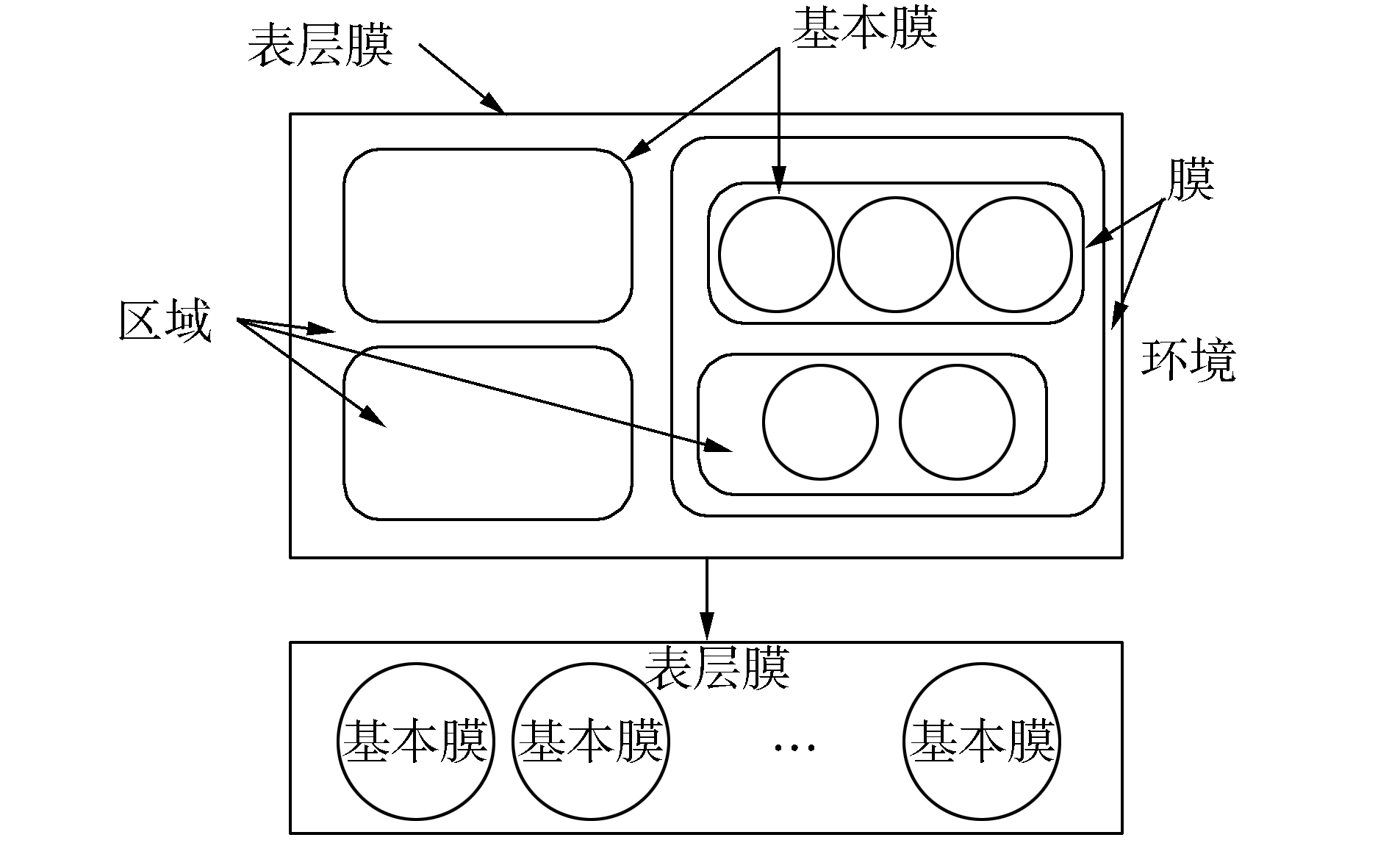图 1 膜系统与其简化结构 Fig.1 Membrane system with its simplified structure
2 P-GA算法

P系统由字符对象多重集、反应规则和膜结构三部分组成。在P系统中，字符对象与多重集分别对应所求多目标优化问题的解和解集，且每一个字符对象都会通过适应度函数得到一个适应度值；反应规则既是算法具体的执行，也有对细胞膜的操作，比如分裂、溶解等；膜结构的运用使算法具有了分布式和并行计算的能力。在每一次的迭代，将随机产生的字符对象放入表层膜区域中，利用表层膜区域中的反应规则和通信规则进行操作；最后通过膜的溶解将多重集释放到表层膜区域中交流信息。为了降低仿真实验难度，所提算法采用的细胞型膜结构只有表层膜与基本膜，这种二层结构相较于原结构复杂度较低。

P-GA算法基于膜计算理论，包含了遗传机制，利用NSGA-Ⅱ算法的非支配排序和拥挤距离两种机制来设计算法，详细步骤如下。

1) 根据优化问题的约束条件，在表层膜的区域内随机生成N个字符对象(Ni≥1)，所有字符对象均为十进制编码，具体形式为

 ${s_{i, j}} = ({s_{{\rm{max}}, j}}-{s_{{\rm{min}}, j}}) \times {\rm{rand}}() + {s_{{\rm{max}}, j}}$ (5)

2) 根据所要优化问题的目标函数计算出每个字符对象的适应度值，从而完成对所有字符对象的评价。

3) 在初始化完成以后，利用表层膜的分裂规则，在表层膜内部区域分裂出m+1个基本膜，且分裂出的基本膜具有求解多目标优化问题的能力。首先，将初始化完成的m个多重集复制一份发送到第m+1个基本膜的区域中；然后，将m个多重集发送到前m个基本膜的区域中，逐一对应。具体表层膜分裂规则如式(6)：

 ${[\;]_0} \to \left[{{{[\;]}_1}, {{[\;]}_2}, \cdots, {{[\;]}_m}, {{[\;]}_{m + 1}}} \right]$ (6)

 $\left\{ \begin{array}{l} \dot w = {\rm{sort}}\left( w \right)\\ \dot w = \{ {w_1}, {w_2}, \cdots, {w_m}\} \\ n = {\rm{sizeof}}\left( w \right)/m \end{array} \right.$ (7)

4) 对前m个基本膜用GA算法中的交叉规则进行多线程并行计算，以获得新的字符对象，而并行计算可以极大地加快求解速度，具体交叉操作为

 $\left\{ \begin{array}{l} {S_{t + 1}}^1 = \alpha \cdot{S_t}^1 + \left( {1-\alpha } \right)\cdot{S_t}^2\\ {S_{t + 1}}^2 = \alpha \cdot{S_t}^2 + \left( {1-\alpha } \right)\cdot{S_t}^1 \end{array} \right.$ (8)

5) 利用通信规则将前m个基本膜中产生的交叉结果复制一份发送到第m+1个基本膜中，对第m+1个基本膜中的多重集进行变异操作。由于自然进化中种群变异率往往比较低，因此在一次迭代中，只有利用足够多的字符对象进行变异操作才能产生更多的新个体，具体变异操作如式(9)所示：

 ${S_{(t + 1, j)}} = {S_{(t, j)}} + 0.1 \times ({S_{({\rm{max}}, j)}}{S_{({\rm{min}}, j)}}) \times {\rm{rand}}(\;\;)$ (9)

6) 当每个基本膜中的规则和操作都结束以后，调用基本膜区域内的溶解规则，当m+1个基本膜全部溶解后，表层膜区域中就会有来自于不同基本膜中的字符对象；本文引入外部档案集，将从基本膜中溶解出的字符对象插入到外部档案集中，而后将表层膜区域中的字符对象与归入外部档案的字符对象进行非支配排序操作。外部档案的执行流程为：①对所有字符对象执行NSGA-Ⅱ算法中的两种机制；②根据执行结果选取支配等级较低的字符对象，若两者支配等级相等，则比较拥挤距离大小，选取拥挤距离较大的字符对象；③通过②的选取后，将获得的所有字符对象归入外部档案集，然后删除档案中受支配的字符对象，剩余存在于外部档案集中的字符对象将作为下一代的非支配多重集。

7) 如果算法不满足条件，则重复2)~6)的步骤；若算法满足条件，则终止迭代，此时将表层膜区域中的多重集输出即可。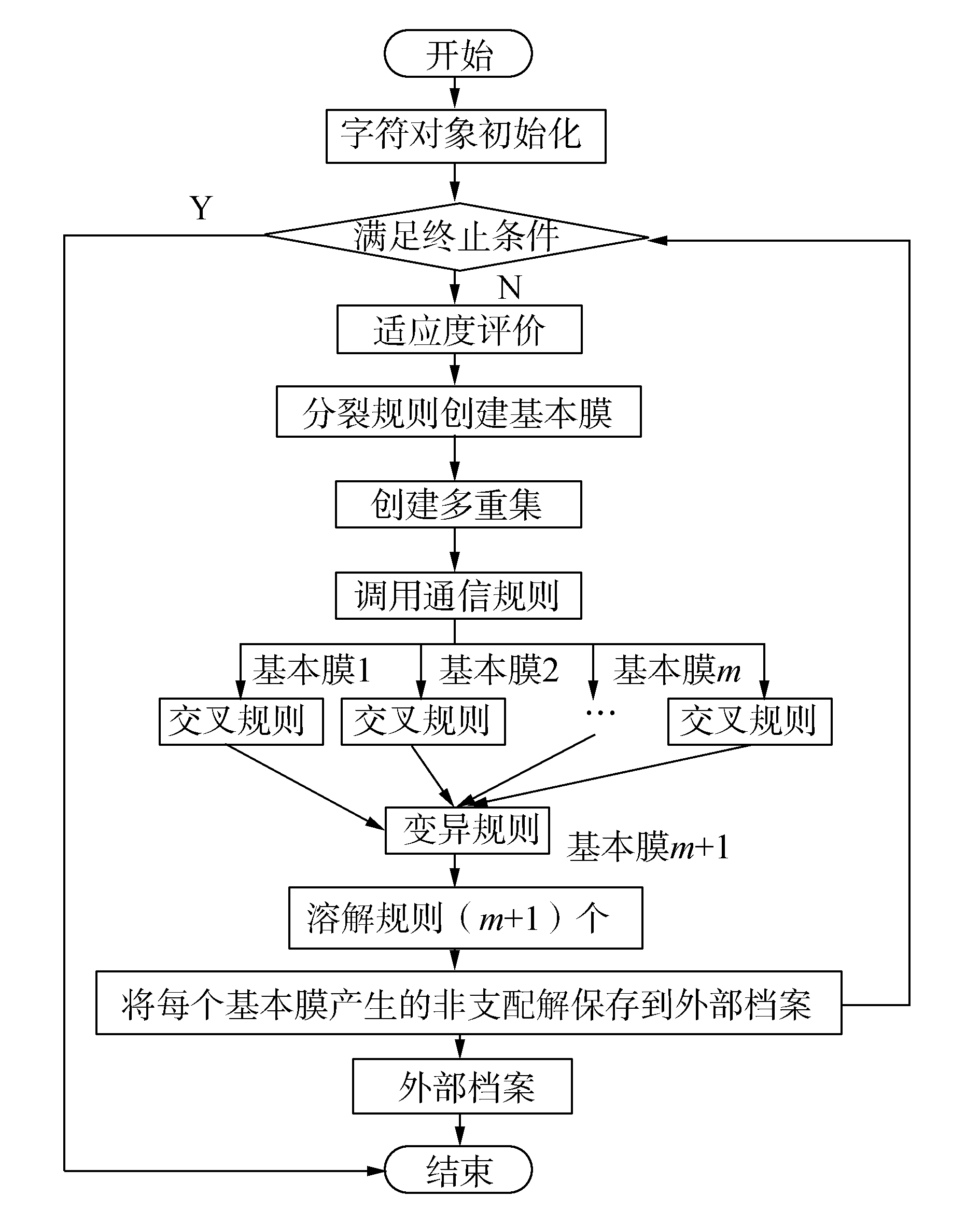图 2 P-GA算法流程图 Fig.2 P-GA algorithm implementation flow chart
3 仿真实验 3.1 测试函数

3.2 实验环境表 1 算法参数设置 Tab.1 Algorithm parameter setting

3.3 仿真结果

KUR测试维度为3，ZDT系列的维度均为30，迭代次数均为100次，测得结果如图 3~6所示。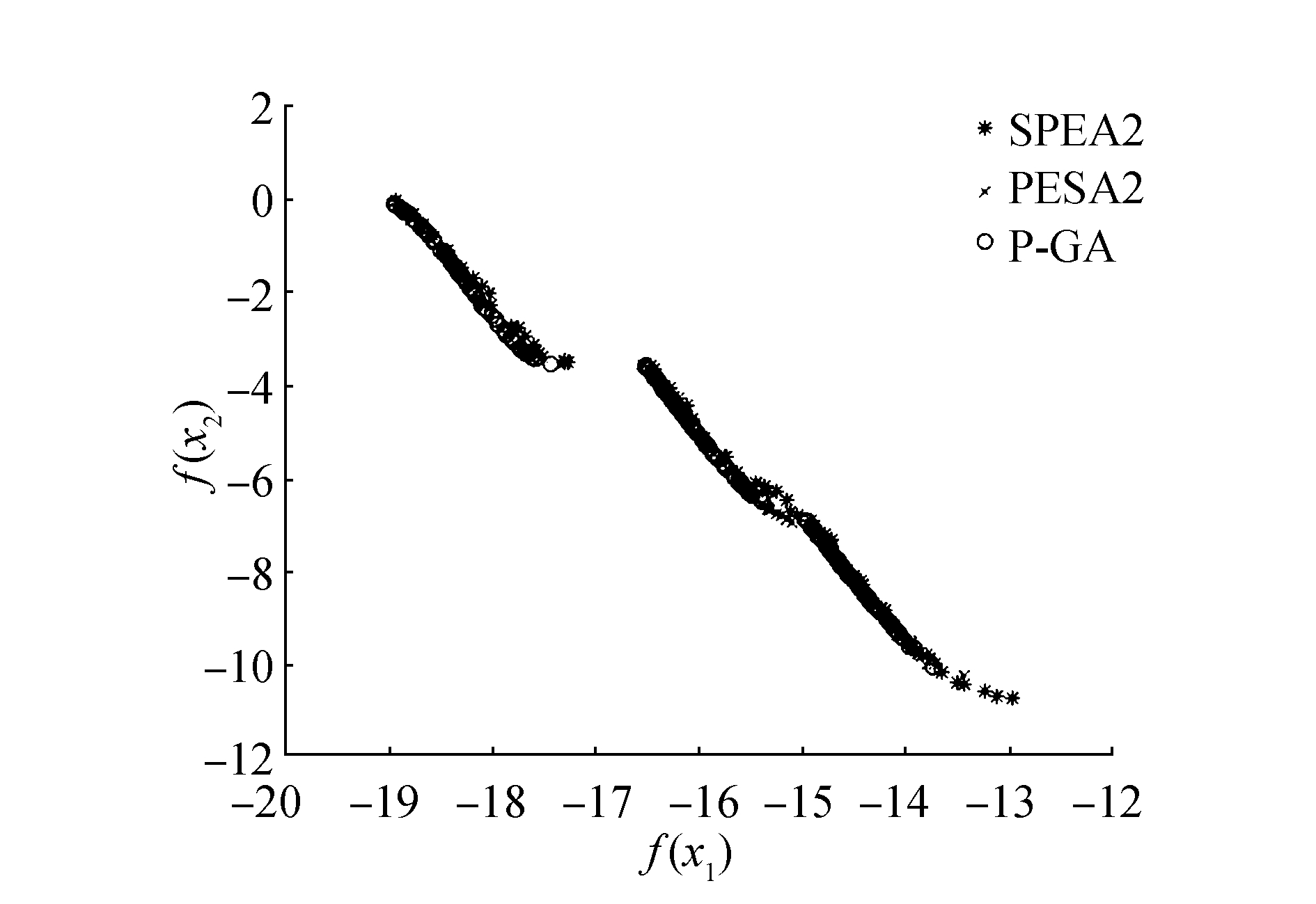图 3 基于KUR测试函数分布图 Fig.3 KUR test function distribution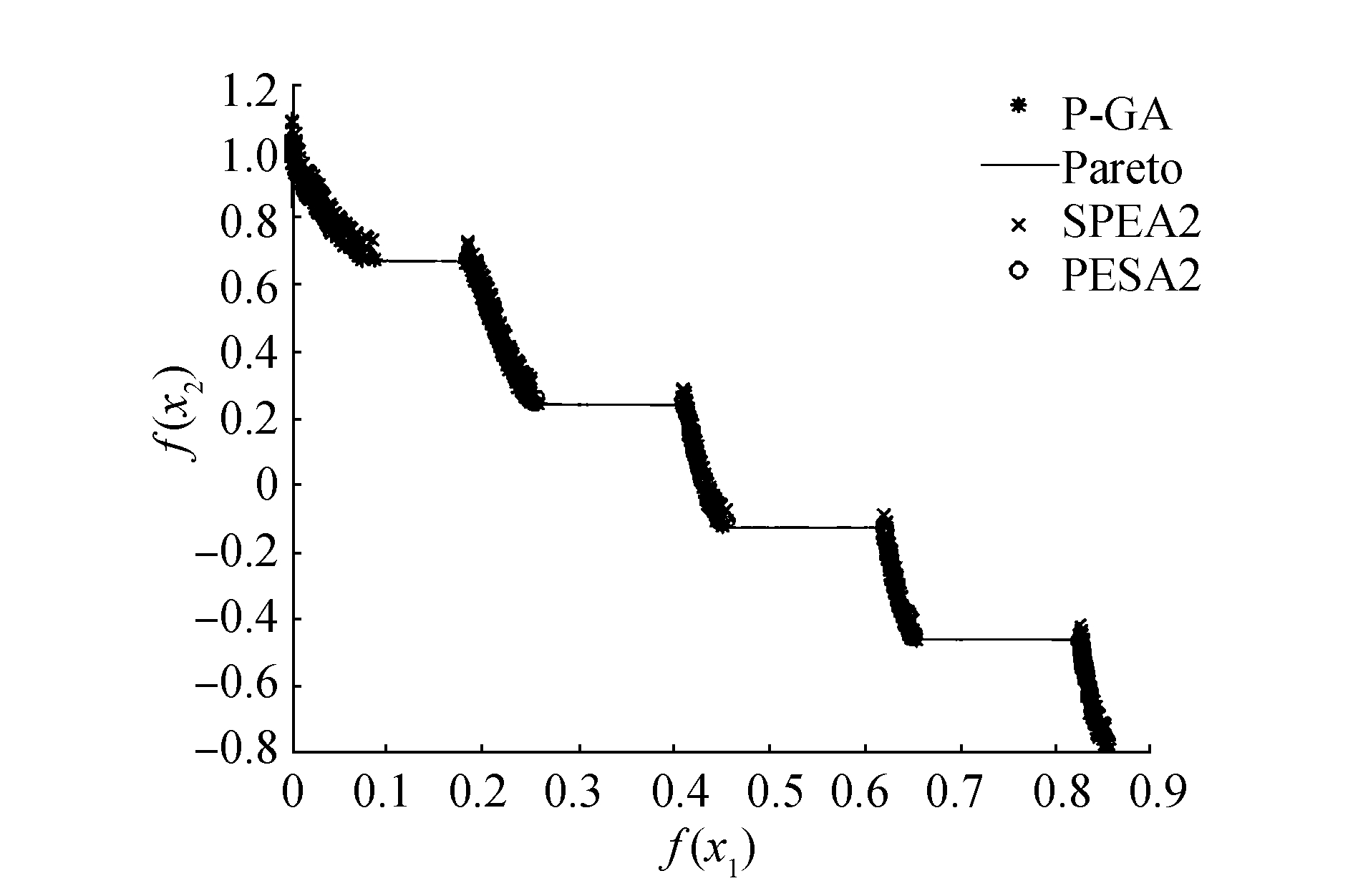图 6 基于ZDT3测试函数分布 Fig.6 ZDT3 test function distribution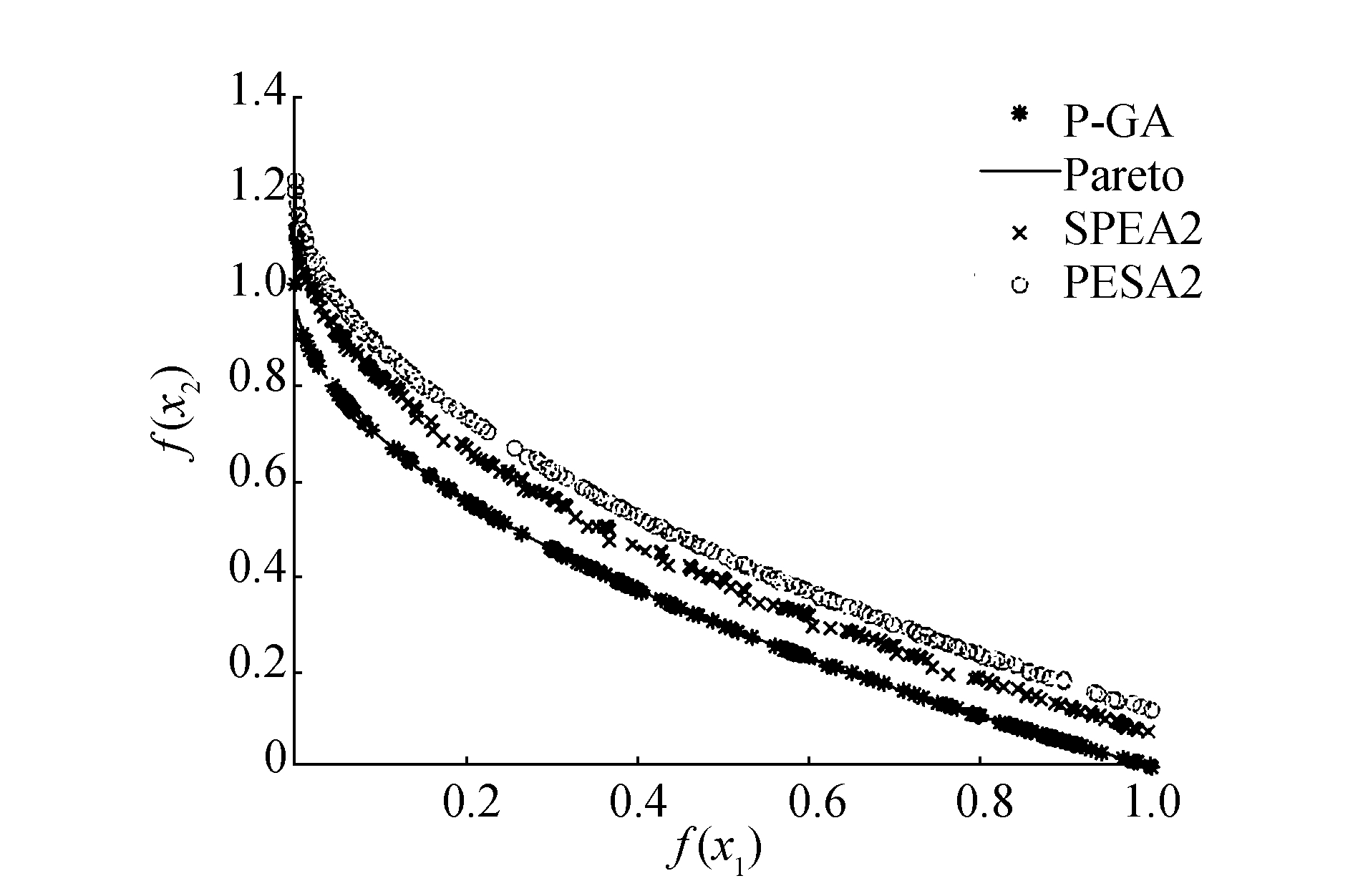图 4 基于ZDT1测试函数分布图 Fig.4 ZDT1 test function distribution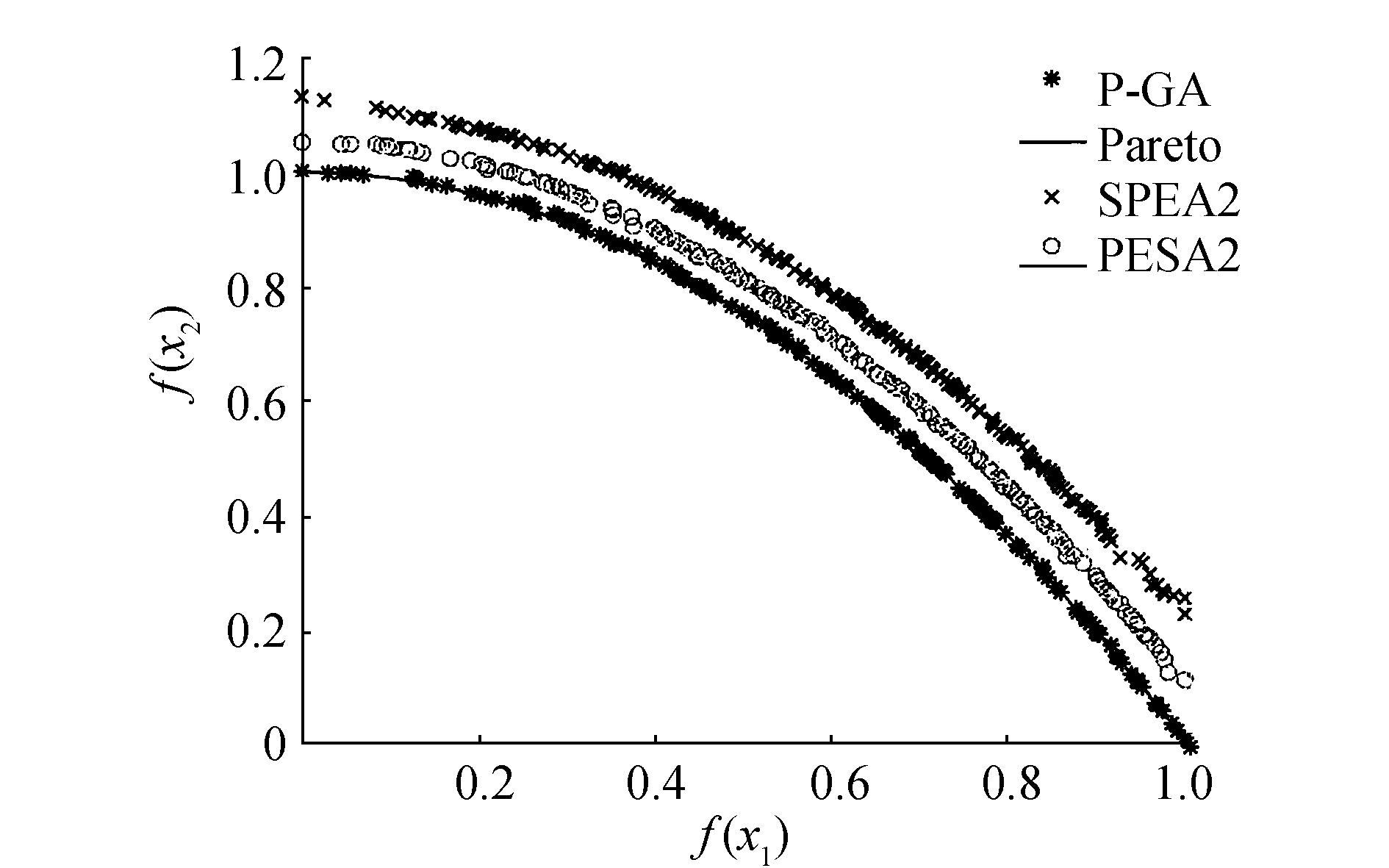图 5 基于ZDT2测试函数分布 Fig.5 ZDT2 test function distribution表 2 3个算法在4个测试函数上的IGD性能统计 Tab.2 Comparison of IGD performance of three algorithms on four test functions

4 结束语

  FONSECA C M, FLEMING P J. Genetic algorithms for multi-objective optimization:formulation, discussion and generalization[C]//The 5th International Conference on Genetic Algorithms. San Mateo, CA, USA, 1993:416-423. (0)  SRINIVAS N, DEB K. Multi-objective optimization using non-dominated sorting in genetic algorithms[J]. Evolutionary computation, 1994, 2(3): 221-248. DOI:10.1162/evco.1994.2.3.221 (0)  HORN J, NAFPLIOTIS N, GOLDBERG D E. A niched pareto genetic algorithm for multi-objective optimization[C]//Proceedings of the first IEEE Conference on Evolutionary Computation. Orlando, FL, USA, 1994:82-87. (0)  ZITZLER E, THIELE L. Multi-objective evolutionary algorithms:a comparative case study and the strength Pareto approach[J]. IEEE trans. on evolutionary computation, 199, 3(4): 257-271. (0)  MARK ERICKSON A M, HORN J. The niched pareto genetic algorithm 2 applied to the design of groundwater remediation systems[J]. Lecture notes in computer science, 2001, 1993: 681-695. DOI:10.1007/3-540-44719-9 (0)  DEB K, PRATAP A, AGARWAL S, et al. A fast and elitist multi-objective genetic algorithm:NSGA-Ⅱ[J]. IEEE trans. on evolutionary computation, 2002, 6(2): 182-197. DOI:10.1109/4235.996017 (0)  PAUN G, ROZENBERG G. A guide to membrane computing[J]. Theoretical computer science, 2002, 287(1): 73-100. DOI:10.1016/S0304-3975(02)00136-6 (0)  ZAHARIE D, CIOBANU G. Distributed evolutionary algorithms inspired by membranes in solving continuous optimization problems[J]. Lecture notes in computer science, 2009, 4361: 536-553. (0)  LIU C, HAN M, WANG X Z. A multi-objective evolutionary algorithm based on membrane systems[C]//Fourth International Workshop on Advanced Computational Intelligence. Wuhan, China, 2011:103-109. (0)  ZHANG G, CHENG J, GHEORGHE M, et al. A hybrid approach based on differential evolution and tissue membrane systems for solving constrained manufacturing parameter optimization problems[J]. Applied soft computing, 2013, 13(3): 1528-1542. DOI:10.1016/j.asoc.2012.05.032 (0)  韩敏, 刘闯, 邢军. 一种基于膜系统理论的多目标演算法[J]. 自动化学报, 2014, 40(3): 431-438. HAN Min, LIU Chuang, XING Jun. A multi-objective evolutionary algorithm based on membrane system theory[J]. Acta automatica sinica, 2014, 40(3): 431-438. (0)  PAUN G, ROZENBERG G, SALOMAA A. The Oxford handbook of membrane computing[M]. Oxford: Oxford University Press, 2010. (0)  张葛祥, 潘林强. 自然计算的新分支——膜计算[J]. 计算机学报, 2010, 33(2): 208-214. ZHANG Gexiang, PAN Linqiang. A survey of membrane computing as a new branch of natural computing[J]. Chinese journal of computers, 2010, 33(2): 208-214. (0)  张葛祥, 程吉祥, 王涛, 等. 膜计算:理论与应用[M]. 北京: 科学出版社, 2015. (0)  张玉良. 改进粒子群优化算法在图像矢量量化中的应用研究[D]. 天津: 天津工业大学, 2013. ZHANG Yuliang. Application of improved particle swarm optimization algorithm in image vector quantization[D]. Tianjin:Tianjin Polytechnic University, 2013. (0)  周明. 遗传算法原理及应用[M]. 北京: 国防工业出版社, 1999. (0)  李俊. 基于膜计算模型的多目标优化算法研究[D]. 合肥: 安徽大学, 2016. LI Jun. Study on Multi-objective Optimization Algorithm Based on Membrane Computing Model[D]. Hefei:Anhui University, 2016. (0)  黄席越, 张著洪, 何传江, 等. 现代智能算法理论及应用[M]. 北京: 科学出版社, 2005. (0)  JU Y, ZHANG S, DING N, et al. Complex network clustering by a multi-objective evolutionary algorithm based on decomposition and membrane structure[J]. Scientific reports, 2016, 6: 33870. DOI:10.1038/srep33870 (0)  ZHANG G, LI Y, GHEORGHE M. A multi-objective membrane algorithm for knapsack problems[C]//IEEE Fifth International Conference on Bio-Inspired Computing:Theories and Applications. Gwalior, India, 2012:604-609. (0)